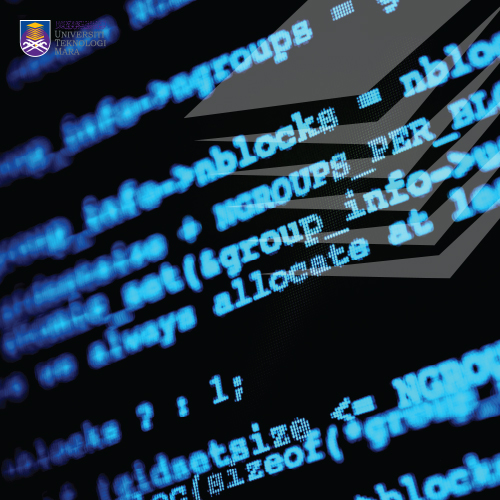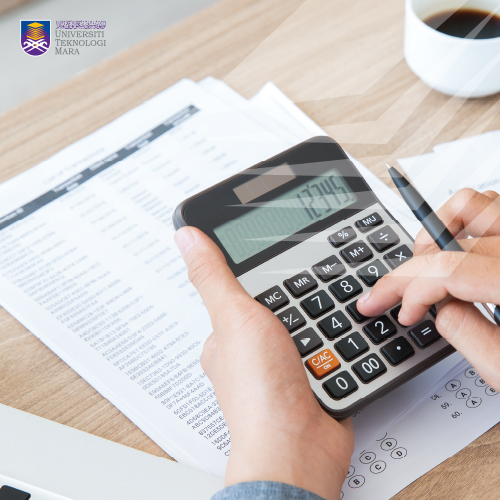• We are available for any custom works this month
• Main office: Springville center X264, Park Ave S.01
• Call us (123) 456-7890 - (123) 555-7891

## Course Information

### FOUNDATION MATHEMATICS II FOR ENGINEERS

•••This course covers wide range of topics in calculus including differentiation, application of differentiation, application of differentiation, series, integration, application of integration, numerical methods, differential equations and conic section. Applications in engineering related problem will also be discussed in certain topics. By the end of this course, students are expected to acquire and apply knowledge of mathematics in various field of engineering.

#### Course Syllabus

The Derivative
- Definition of Limit, Computing limits (Including End Behavior)
- Continuity
- Definition of Derivative
- Techniques of Differentiation
- Derivative of Trigonometric Functions
- Derivative of Exponential Functions
- Derivative of Logarithmic Functions
- Implicit Differentiation

Applications of Derivative
- Tangent and Normals
- Rate of Change and Related Rates
- Curve Sketching: Polynomial Function (Up to Third Degree)

Series
- Power Series
- Taylor Series
- Maclaurin Series
- Binomial Series

Integration
- Indefinite Integral
- Rules of Integration
- Integration of exponential, logarithmic and trigonometric functions
- Integration by substitution, by parts and partial fraction methods

Application of Integration
- Definite Integral
- Area between two curves
- Volume of solid of revolution
- Moment of Inertia

Numerical Methods
- Trapezoidal Rule
- Newton-Raphson Method

Differential Equations
- First Order Separable Differential Equation
- First Order Linear Equation
- Applications of First Order Differential Equation

Conic Section
- Circle
- Parabola
- Ellipse